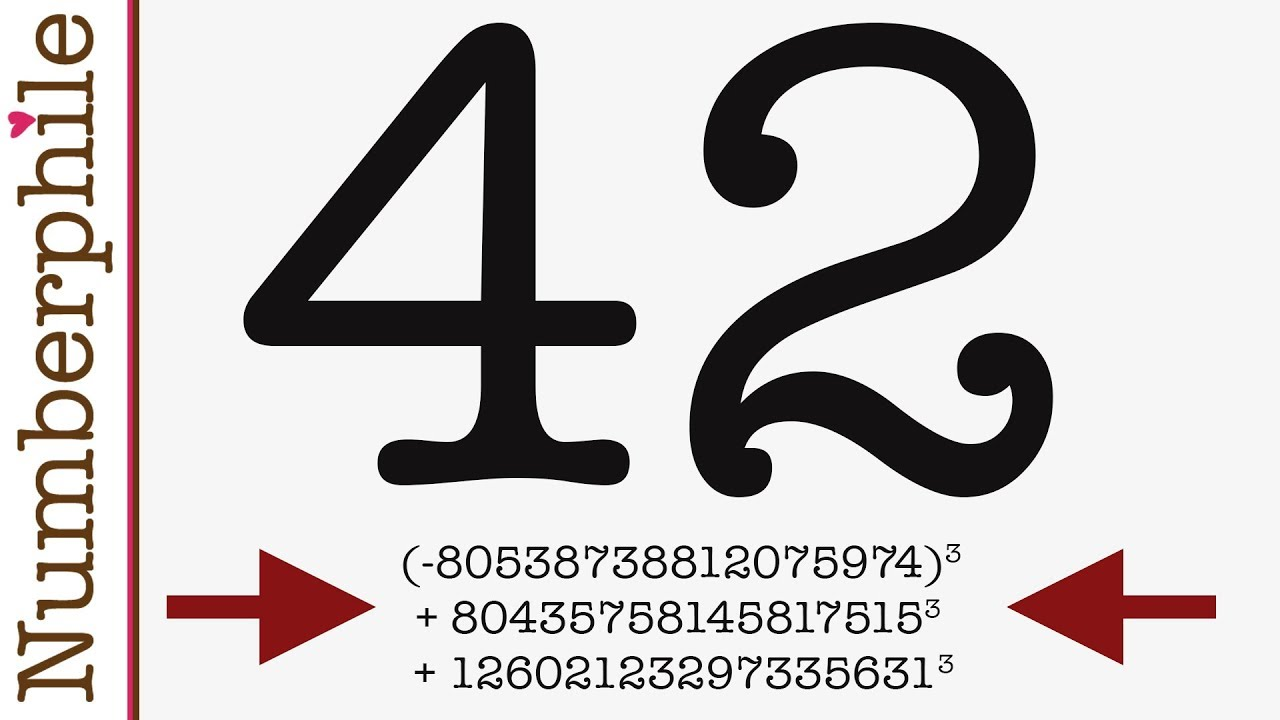Uncategorized

# Mathematicians Solve an Enduring ’42’ Problem Using Planetary SupercomputerMathematicians have cracked a math puzzle that’s stumped computers and humans alike for 65 years: How can the number 42 be expressed as the sum of three cubed numbers?

While it might seem simple on its face, this question is part of an enduring number-theory conundrum that goes back to at least 1954 and may have been mulled over by Greek thinkers as early as the third century. The underlying equation to solve looks like this:

x^3 + y^3 + z^3 = k

K is each of the numbers from 1 to 100; the question is, what are x, y, and z?

This is an example of a Diophantine equation, named for the ancient mathematician Diophantus of Alexandria, who proposed a string of similar equations with multiple unknown variables about 1,800 years ago.

But slowly, over many years, each value of k was eventually solved for (or proved unsolvable), thanks to sophisticated techniques and modern computers — except the last two, the most difficult of all; 33 and 42.

Fast forward to 2019 and Professor Andrew Booker’s mathematical ingenuity plus weeks on a university supercomputer finally found an answer for 33, meaning that the last number outstanding in this decades-old conundrum, the toughest nut to crack, was that firm favorite of Douglas Adams fans everywhere.

So, we were left with the hardest one of them all: 42. This proved a much more obstinate problem, so Booker enlisted the aid of fellow MIT mathematician Andrew Sutherland, an expert in massively parallel computation.

Professors Booker and Sutherland’s solution for 42 would be found by using Charity Engine, a ‘worldwide computer’ that harnesses idle, unused computing power from over 500,000 home PCs to create a crowd-sourced, super-green platform made entirely from otherwise wasted capacity.

The answer, which took over a million hours of calculating to prove, is as follows:

X = -80538738812075974

Y = 80435758145817515

Z = 12602123297335631

So, the full equation is (-80538738812075974)3 + 804357581458175153 + 126021232973356313 = 42.

And with these almost infinitely improbable numbers, the famous Solutions of the Diophantine Equation (1954) may finally be laid to rest for every value of k from one to 100 — even 42. “I feel relieved,” Booker mentioned.

Besides, they did an enjoyable reveal of their success: based on The Aperiodical, each mathematician quietly modified their private web sites to the answer, and named the pages “Life, the Universe, and all the things,” a becoming nod to Douglas Adams.

But it’s not yet over because if one goes up an order of magnitude to 1,000, there are nonetheless loads of numbers to resolve – 114, 165, 390, 579, 627, 633, 732, 906, 921 and 975 are all awaiting an answer to the sum of three cubes.

Close

Close